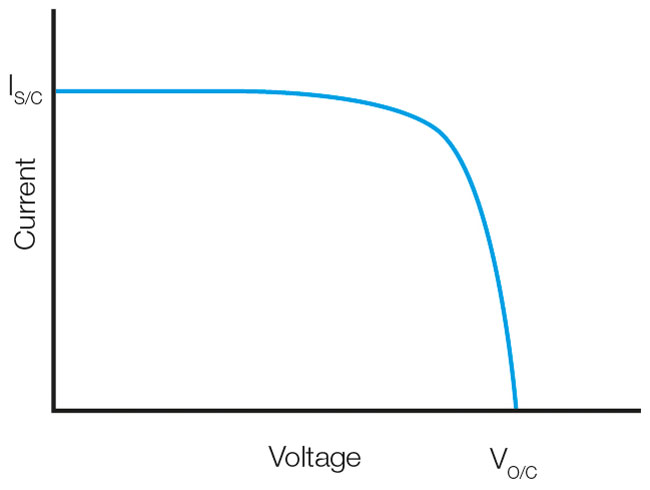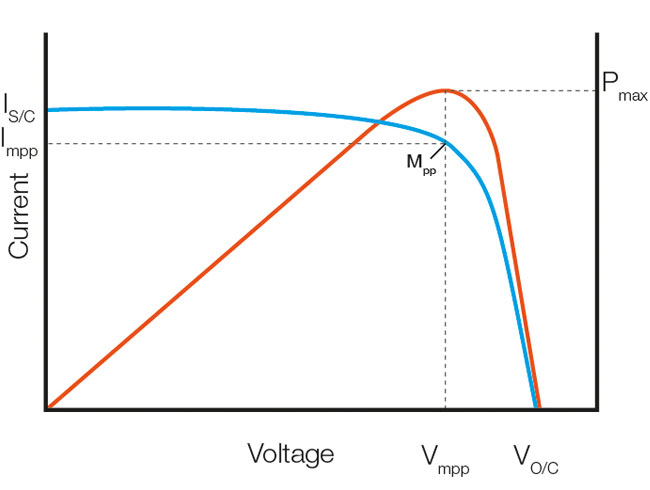The electrical power produced by a solar PV cell or module is a function of the current (I) and voltage (V) characteristics. Measuring the relationship between current and voltage whilst varying the electrical load connected to the PV cell or module from open circuit to short circuit produces a characteristic current vs voltage (I-V) curve as shown below in figure 1. The points where the curve meets the current and voltage axis are the short circuit current Is/c and open circuit voltage Vo/c respectively.Power is the product of voltage and current and so the power vs voltage curve shown in figure 2 can be generated from the measured voltage and current data. The power vs voltage curve shows the point at which the power is a maximum (Pmax). The corresponding maximum power point Mpp is shown on the I-V curve. Loading the PV module such that the current is Impp and voltage is Vmpp will operate the PV module at the maximum power point (Mpp) and result in the maximum power generation.

### Still need help? Ask us.

Stay in the know
Sign up to our mailing list today to stay up to date with the latest industry news and information from Seaward.

### I'd like to talk to a sales advisor